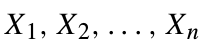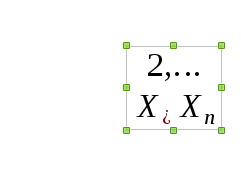# How to type the mathematic series x_1,x_2,...,x_3 in Writer formula?

There is a very typical math series that I want to type in Writer’s formula. It is as follows:To do this, I write X_1, X_2, …,X_n, which results in the the following result:I also, tried ““X_1,X_2,…,X_n””, `X_1,X_2,…,X_n`, and {X_1,X_2,…,X_n}, none of which worked. How should I type that?

Make sure that there’s a space between de index and the next comma: X_1 , X_2 ,…,X_n to “tell”" Libre that the comma doesn’t belong to the index. Braces also work: X_{1}, X_{2},…,X_n and give a neater result.

Thanks, my mistake was in spaces. But, I tried removing space between comma and the next number i.e. X_1, X_2, …, X_n and it worked. Removing space between comma and index (as you said) also works.